### Home > A2C > Chapter 10 > Lesson 10.1.2 > Problem10-46

10-46.
1. Antonio and Giancarlo are playing a board game that uses three spinners, which are shown in the diagrams below. The first is two-thirds red and one-third green. The second is all red and split into four equal parts with the numbers 0, 2, 4, and 6 in each quarter. The third is all green and split into three unequal sections, marked with a 6, a 4, and a 2. On each turn the player has to spin two of the spinners to determine how far he gets to move his marker. The color that comes up on the first spinner determines which of the other two spinners the player has to spin to get the number of spaces to move. Homework Help ✎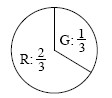Spinner #1 Red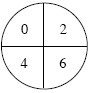Spinner # 2 Green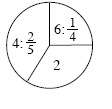Spinner #3
1. Make an area model or tree diagram to represent the possible outcomes.

2. What is the probability that Giancarlo will get to move his marker 4 or 6 spaces?

3. What is the probability he will have to stay put?

4. What is the probability that he will get to move?

5. Antonio was moving his marker 2 spaces. What is the probability that he had spun green on the first spinner?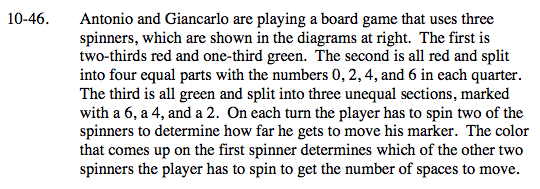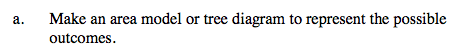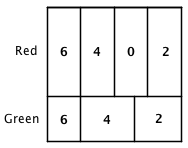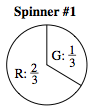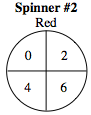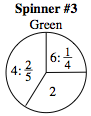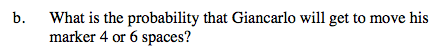Place probabilities on the appropriate sides and multiply to find the probabilities of the seven possible outcomes.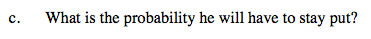$\frac{2}{3}\cdot\frac{1}{4}=\frac{1}{6}$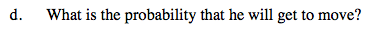$1-\frac{1}{6}=\frac{5}{6}$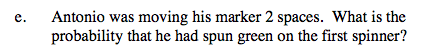Divide the probability of getting green and then 2 by the probability of getting 2 with either color.